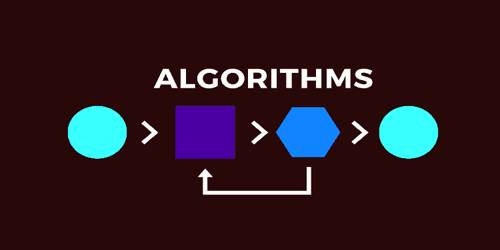# Characteristics of an Algorithm

An algorithm is a well-defined list of steps for solving a particular problem. One major purpose of this text is to develop an efficient algorithm for the processing of our data. The time and space it uses are two major measures of the efficiency of an algorithm. A search engine algorithm, for example, takes search strings of keywords and operators as input, searches its related database for relevant web pages, and returns results.

The characteristics of a good algorithm are:

(a) Precision: the steps are precisely stated.

(b) Uniqueness: the results of each step are uniquely by defined and only depended on the input and result of the proceeding steps.

(c) Finiteness: the algorithm stops after a finite number of instructions are executed.

(d) Input: the algorithm receives input.

(e) Output: the algorithm produces output.

(f) Generality: the algorithm applies to a set of inputs.

(g) Effectiveness: An algorithm is also generally expected to be effective. This means that all of the operations to be performed in the algorithm must be sufficiently basic that they can in principle be done exactly and in a finite length of time.